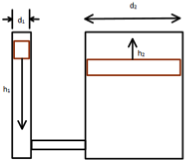# 2.1: Pascal's Law

$$\newcommand{\vecs}{\overset { \rightharpoonup} {\mathbf{#1}} }$$ $$\newcommand{\vecd}{\overset{-\!-\!\rightharpoonup}{\vphantom{a}\smash {#1}}}$$$$\newcommand{\id}{\mathrm{id}}$$ $$\newcommand{\Span}{\mathrm{span}}$$ $$\newcommand{\kernel}{\mathrm{null}\,}$$ $$\newcommand{\range}{\mathrm{range}\,}$$ $$\newcommand{\RealPart}{\mathrm{Re}}$$ $$\newcommand{\ImaginaryPart}{\mathrm{Im}}$$ $$\newcommand{\Argument}{\mathrm{Arg}}$$ $$\newcommand{\norm}{\| #1 \|}$$ $$\newcommand{\inner}{\langle #1, #2 \rangle}$$ $$\newcommand{\Span}{\mathrm{span}}$$ $$\newcommand{\id}{\mathrm{id}}$$ $$\newcommand{\Span}{\mathrm{span}}$$ $$\newcommand{\kernel}{\mathrm{null}\,}$$ $$\newcommand{\range}{\mathrm{range}\,}$$ $$\newcommand{\RealPart}{\mathrm{Re}}$$ $$\newcommand{\ImaginaryPart}{\mathrm{Im}}$$ $$\newcommand{\Argument}{\mathrm{Arg}}$$ $$\newcommand{\norm}{\| #1 \|}$$ $$\newcommand{\inner}{\langle #1, #2 \rangle}$$ $$\newcommand{\Span}{\mathrm{span}}$$$$\newcommand{\AA}{\unicode[.8,0]{x212B}}$$A YouTube element has been excluded from this version of the text. You can view it online here: https://openoregon.pressbooks.pub/hydraulics/?p=41

Describe Pascal’s Law and the formula used to relate force, pressure, and area. Solve for F in terms of p and A. Solve for p in terms of F and A. Solve for A in terms of F and p.

List common units of force, pressure, and area.

Determine equivalency for psi, Pa, and bar.

If area is kept constant determine how changes in pressure affect force.

If pressure is kept constant determine how changes in area affect force.

If area is kept constant determine how changes in force affect pressure.

If force is kept constant determine how changes in area affect pressure.Given a force multiplication system with the following dimensions:

d1 = 3/4 in

F1 = 120 lbf

h1 = 4 in

d2 = 1 ¼ in

Calculate A1, p, V1, A2, F2, h2, energyin, energyout

Comment on the differences between the cap end and rod end of a double acting cylinder with respect to functional area.

Explain why the pressure necessary to retract is greater than the pressure necessary to extend with the same force for a double acting cylinder.

Explain why the force of extension is greater than the force of retraction given the same pressure limit for a double acting cylinder.

Given cylinder X with the following dimensions:

dcap = 2 7/8 in

drod = 7/8 in

Calculate pext and pret for cylinder X given the system is tasked with moving a 1350lbf object.

Calculate FextMAX and FretMAX for cylinder X given the system is limited to 640psi.

This page titled 2.1: Pascal's Law is shared under a CC BY-NC 4.0 license and was authored, remixed, and/or curated by Jim Pytel (OpenOregon) via source content that was edited to the style and standards of the LibreTexts platform; a detailed edit history is available upon request.